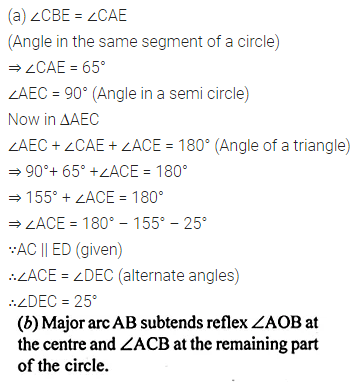Guru

# Question 9. (a) In the figure (i) given below, chord ED is parallel to the diameter AC of the circle. Given ∠CBE = 65°, calculate ∠DEC.

• 0

This is circle based question from Chapter name- circles
Topic – Angle properties of circles
Chapter number- 15

In this figure, we have been given the certain information about the circles and we have to find the value of <DEC

ICSE Avichal publication
Understanding ICSE Mathematics
Question no 9(a)

Share